## A SAS Macro for Dynamic Page Breaks

Started ‎03-15-2021 by
Modified ‎05-13-2021 by
Views 1,876
Paper 1108-2021
Authors

Manohar Modem, Cytel Inc; Bhavana Bommisetty, Vita Data Sciences

## Abstract

In clinical domain, we usually create many safety and efficacy tables with various statistics. While creating these tables, we introduce SAS dataset with statistics into PROC REPORT to create listing or rtf output. Using PROC REPORT-BREAK-PAGE, we can make sure that each parameter statistics starts in a new page in the output. If we want to make sure that a group of statistics does not break abruptly between pages, we may need to use conditional statements to assign page numbers. Whenever there is an update in mock shell or data, the number of rows in the output may increase or decrease, which in turn requires an update in conditional statements to prevent abrupt, breaks in the output. This led to an effort to create a macro, which prevents abrupt page breaks and provides meaningful page numbers. This paper describes how the page numbers were dynamically assigned using SAS macro.

## Introduction

To create a table, we generate descriptive or inferential statistics using various SAS procedures and present them as per mock shell. We introduce the final dataset, which contains all the statistics in the required format into PROC REPORT procedure to create rtf output. In most cases, we may have to create a custom variable using IF-THEN-ELSE conditional statements and introduce this in PROC REPORT-BREAK-PAGE to avoid abrupt breaks in the output.

Figure 1 and Figure 2 shows an abrupt break in a demographics table output where ‘Height’ statistics are shown in two different pages, as page 1 cannot accommodate any more rows. Figure 3 and Figure 4 shows that ‘Height' statistics are pushed to page 2. We can do this by creating variable using IF-THEN-ELSE conditional statements and using this variable in PROC REPORT.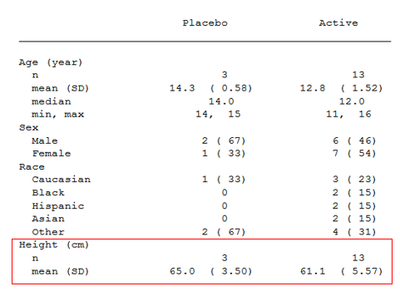### Figure 1. Demographics table - Page 1 of 2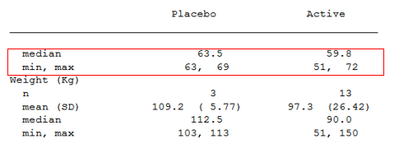### Figure 2. Demographics table -  Page 2 of 2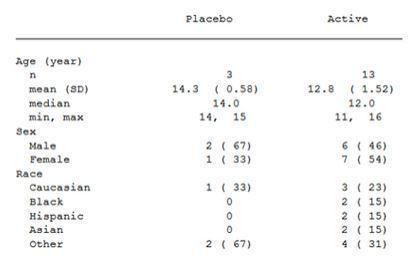### Figure 3. Demographics table - Page 1 of 2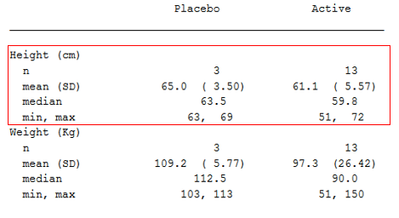### Figure 4. Demographics table - Page 2 of 2

In clinical domain, there are different kinds of table like demographic, lab shift, change from baseline etc., which have different shell structure.  So, for each of these tables, you may need to create a custom variable using IF-THEN-ELSE conditional statements to avoid abrupt page breaks. This led to an effort to create %pageno macro that can be used across various tables.

## %PAGENO Macro description

The functionality of this macro is to create a numeric variable, which prevents abrupt page breaks in the output.

This macro contains seven keyword macro parameters.

• &indata = name of the input dataset. By default, this macro considers the latest created dataset before the macro call.
• &outdata = name of the output dataset. By default, this macro creates an output dataset named “pg_final”.
• &mxlnpg = maximum number of rows desired per page in the rtf or listing output.
• &grpvars = list of grouping variables separated by space. It can be one to many character or numeric variables. A variable can be given only once in its numeric or character form. Eg: If variable 'AVISITN' is just a numeric representation of  'AVISIT', then use only one them in &grpvars.
• &statvarc = variable with row labels along with its corresponding length in proc report separated by comma.
• &colsdlm = list of character variables and delimiter separated by comma.
• &debug = possible values are 0 and 1. Default value is 0. &debug=1 generates all the intermediate datasets.

&mxlnpg, &grpvars and &statvarc are the three macro parameters that are mandatory for each call. We can use other parameters as and when required.

Usually in a table rtf output, each page consists of three parts - Title, Footnotes and Body. The number of lines occupied by title and footnotes varies from one table to another. As a result, the number of lines available in the body is different for various kind of tables.

Let us see few scenarios to understand the functionality of %pageno macro.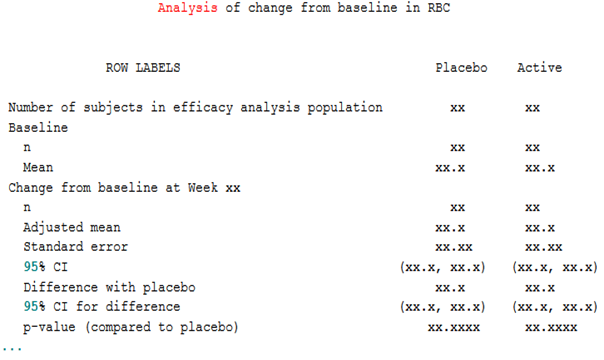Figure 5. Mock shell

Figure 5 is an example of a table mock shell. All the  following scenarios were created based on this shell.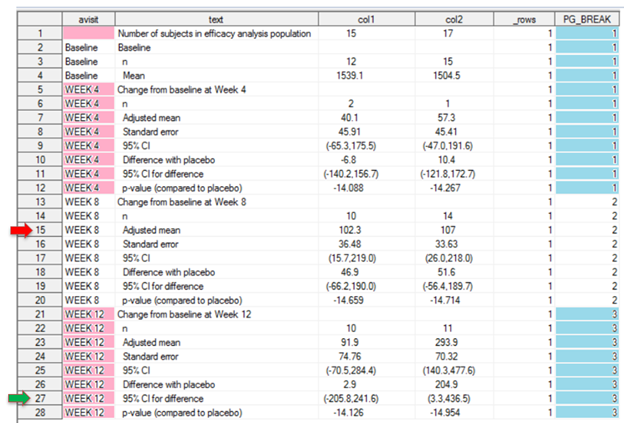Figure 6. Scenario 1

``%pageno(grpvars= avisit, statvarc= %str(text,55), mxlnpg=15);``

Explanation:

• 'AVISIT' in &grpvars is the grouping variable. List of records with same 'AVISIT' variable value represents a section. 'TEXT' in &statvarc is the variable with row labels and 55 is the width given for this variable in proc report. 15 in &mxlnpg is the maximum number of lines we want to see per page with out abrupt page breaks. As the amount of space occupied by titles and footnotes varies from one table to another, we can't have a specific value for &mxlnpg. We need to adjust this &mxlnpg value as needed.
• First macro check occurs at 15th record which is represented by red arrow. If the page break happens after this record, 'WEEK 8' records would be split in two pages which is not desired. As 15th record isn't the last record of 'WEEK 8' records, macro assigns 'PG_BREAK' variable value as 1 for records until AVISIT = 'WEEK 4'.
•  Second check start from the first record of AVISIT = 'WEEK 8'. Next 15th record from first record of AVISIT = 'WEEK 8' is at the record with green arrow. As this record isn't the last record of AVISIT = 'WEEK 12' records, macro assigns 'PG_BREAK' variable value as 2 for records in AVISIT = 'WEEK 8'.
•  Third check starts at the first record of AVISIT = 'WEEK 12'. As there are less than 15 records in this section, macro assigns 'PG_BREAK' variable value as 3.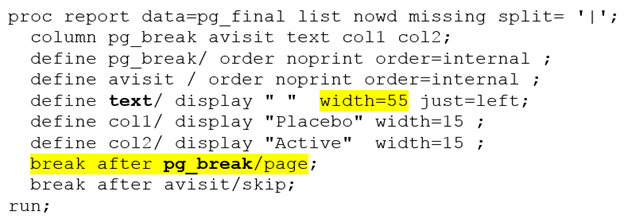Figure 7. Proc report break page

• %pageno macro call generated 'PG_BREAK' variable. We need to  introduce this variable in PROC REPORT- BREAK AFTER - PAGE to prevent abrupt page breaks. Also, notice that  the highlighted text 'width =55' is the value that we used in &statvarc.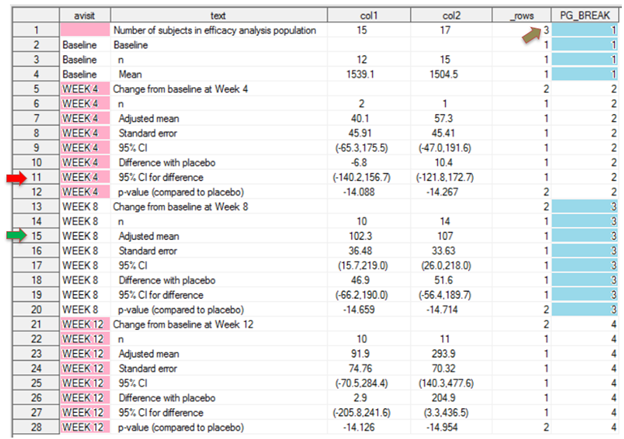Figure 8. Scenario 2

``%pageno(grpvars= avisit, statvarc=%str(text, 25), mxlnpg=14);``
Explanation:
• Macro call in scenario 2 is similar to scenario 1 except for one difference. The width assigned for 'TEXT' variable is 25 which is less when compared to scenario 1 where it was 55. There might be many treatment columns in a study, and we may have to decrease the width assigned for 'TEXT' column. As a result, 'TEXT' value in each observation may wraps to many rows. This macro takes this into consideration and calculates how many rows are needed in the rtf output for each observation in dataset based on width assigned for 'TEXT' variable.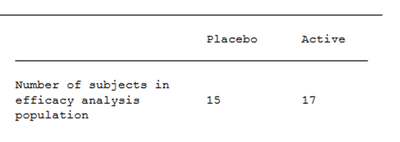Figure 9. Text Wrap

•  '_ROWS'  variable value represents how many rows are needed for that observation in the rtf output. For example, first observation requires three rows in the rtf output as shown in Figure 9.
• &mxlnpg value is 14. First macro check doesn't occur at 14th observation. Instead, it occurs at 11th record which is represented by red arrow. This is because some of the observations require more than 1 row in the rtf output. At 11th record, sum of '_ROWS' count is 14. As a result, first macro check occurs here. As this isn't the last record of AVISIT = 'WEEK 4', macro assigns 'PG_BREAK' value as 1 for records up to AVISIT = 'Baseline'.
• Second check starts from the first record of AVISIT = 'WEEK 4'. Next 14th row from first record of AVISIT = 'WEEK 4' is at green arrow. As this record isn't the last record of AVISIT = 'WEEK 8' records, macro assigns 'PG_BREAK' variable value as 2 for records in AVISIT = 'WEEK 4'. In this way, the check continues until the end of the dataset.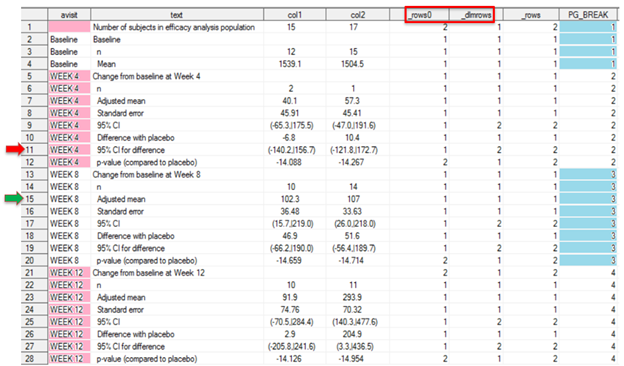Figure 10. Scenario 3

``%pageno(grpvars= avisit, statvarc= %str(text,30), mxlnpg=14, colsdlm=%str(col1 col2, |));``
Explanation:
• Sometimes, we may need to split the values using a delimiter in rtf output.  &colsdlm refers to the list of variables with a delimiter.
• Variables 'COL1' and 'COL2' has symbol '|' as delimiter at records where variable 'TEXT' contains the string "95% CI". For these records, macro assigns 2 rows which is mentioned under the variable '_DLMROWS'.
•  '_ROWS0' is the number of rows assigned for the records based on the width assigned for 'TEXT' variable.
•  Maximum value of '_ROWS0' and '_DLMROWS' is assigned to '_ROWS' which represents the number of rows assigned in the rtf output for an observation.
•  'PG_BREAK' variable is created based on &mxlnpg value and '_ROWS' variable as explained in Scenario 2.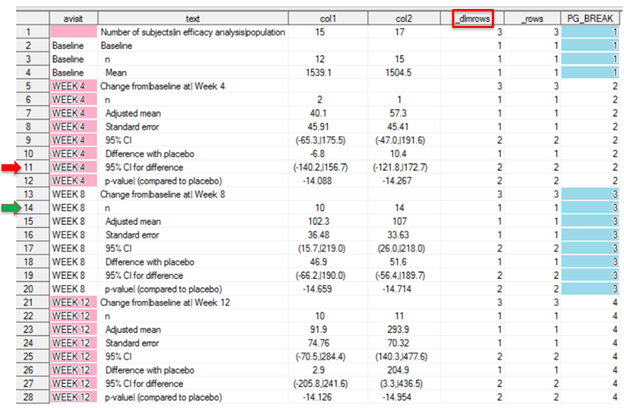Figure 11. Scenario 4

``````%pageno(grpvars= avisit, statvarc= %str(text,30), mxlnpg=17, colsdlm=%str(text col1 col2, |));
``````

Explanation:

• In this scenario, along with 'COL1' and 'COL2', &colsdlm also includes 'TEXT' variable. When 'TEXT' variable is included in &colsdlm, then assignment of rows for an observation is going to be based only on delimiters. Width assigned for 'TEXT' variable is not taken into consideration in assigning rows.
• '_DLMROWS' assigns the maximum number of rows based on the number of delimiters in a value for the list of columns with a delimiter. In an observation, if  the number of delimiters in 'TEXT', 'COL1' and 'COL2' variables are 3, 1 and 2 respectively, then '_ROWS' value would be 4. It indicates that this record requires 4 rows in the rtf output.
•  'PG_BREAK' variable is created based on &mxlnpg value and '_ROWS' variable as explained in Scenario 2.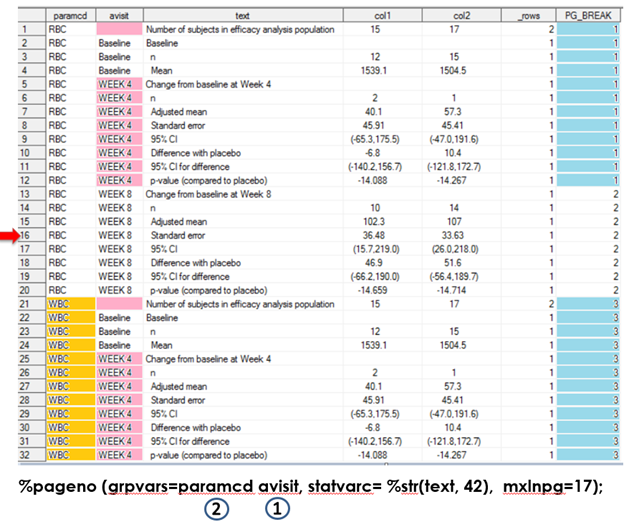Figure 12. Scenario 5

Explanation:

• In this call, there are two variables under &grpvars. These two variables are annotated with values 1 and 2 starting from right to the left.
• Macro check and assignment of 'PG_BREAK' values happens within each value of variable at position 2.
• In this scenario, first macro check occurs at red arrow. As this is not the last record of AVISIT = 'WEEK 8', macro assigns 'PG_BREAK' value as 1 for records until AVISIT = 'WEEK 4'.
• Second macro check starts from first record of AVISIT = 'WEEK 8'. Macro doesn't count the rows from one 'PARAMCD' value to the next. As a result, all 'WEEK 8' records in PARAMCD = 'RBC'  has 'PG_BREAK' value as 2.
• Third check starts from the first record of PARAMCD = 'WBC'. Sum of '_ROWS' values in PARAMCD = 'WBC' is less than 17. So, macro assigns 'PG_BREAK' as 3 for these records.

## Conclusion

By using this macro, we do not need to worry about abrupt breaks in the output either in the first-run or for every data update. This could be quite useful when the table output is too long or when table mock shell is complex with different number of rows in each section.

## REferences

Jensen, ErikLund. "How to split a variable into 200 Character without chopping a word dynamically." Accessed April 15, 2021. https://communities.sas.com/t5/SAS-Programming/How-to-split-a-variable-into-200-Character-without-ch...

## Contact Information

Your comments and questions are valued and encouraged. Contact the author at:

Manohar Modem

Sr. Statistical Programmer

Cytel Inc

Waltham, MA

Email: manohar.modem@gmail.com

Bhavana Bommisetty

Statistical Programmer

Vita Data Sciences

Waltham, MA

SAS and all other SAS Institute Inc. product or service names are registered trademarks or trademarks of SAS Institute Inc. in the USA and other countries. ® indicates USA registration.

Other brand and product names are trademarks of their respective companies.

## APPENDIX

``````%macro pageno (indata=_last_, outdata=pg_final, mxlnpg= , grpvars= , debug=0, statvarc= , colsdlm= );

%local grpvars_1 grpvars_2 grpvars_cs statvarc2 ;
%let grpvars_1 = %scan(&grpvars, -1, " ");
%put grpvars_1 = **&grpvars_1**;
%let grpvars_2 = %scan(&grpvars, -2, " ");
%put grpvars_2 = **&grpvars_2**;
%if %bquote(&grpvars_2) = %str( ) %then %do;
%let grpvars_2= __cat;
%put grpvars_2 = **&grpvars_2**;
%end;
%let grpvars_cs = %qsysfunc(translate(%qsysfunc(compbl(%qsysfunc(strip(&grpvars)))), ",", " "));
%put grpvars_cs = **&grpvars_cs**;
%let statvarc2 = %qsysfunc(strip(&statvarc));
%put statvarc2 = **&statvarc2**;
%if %bquote(&colsdlm) ^= %str( ) %then %do;
%let _dlmvars= %scan(&colsdlm, 1, ",");
%let _dlmval= %left(%trim(%scan(&colsdlm, 2, ",")));
%end;
/*check to see if the input dataset already has "PG_BREAK" variable. If exists, then it is deleted*/
proc sql noprint;
create table __in as
select *
from &indata
;
select count(*) into :varchk
from sashelp.vcolumn
where upcase(libname) = 'WORK' and upcase(memname) = "__IN" and upcase(name) = "PG_BREAK";
quit;

data __dset00 ;
set __in 	%if &varchk ^=0 %then %do;
%str((drop = pg_break _rows ))
%end;;
__lbln=_n_;
run;
/* Find number of rows required in listing or rtf output for text in each line of %nrbuqote(&statvarc) variable*/
data __dset01;
set __dset00;
length nystr \$1000;
str0= %scan(&statvarc2, 1, ",");
_width= %scan(&statvarc2, 2, ",");
_indent= lengthn(trim(str0))-lengthn(strip(str0));
_width2=_width-_indent;
if str0> ' ' then do;
%if &colsdlm ^=  %then %do;
str= translate(str0, ' ', "%qsysfunc(compress(&_dlmval.))");
%end;
%else %do;
str=str0;
%end;
str = strip(compbl(str));
do i = 1 to countw(str,' ');
word = scan(str,i,' ');
if length(nystr) + length(word) + 1 > _width2 then do;
output;
nystr = word;
end;
else nystr = catx(' ',nystr,scan(str,i,' '));
end;
if nystr ne '' then output;
end;
if str0= ' ' then output;
run;

proc sort;
by __lbln;
run;

data __dset02;
set __dset01;
by __lbln;
if first.__lbln then _rows0=1;
else _rows0+1;
if last.__lbln;
keep __lbln _rows0;
run;
/*Find the maximum number of rows required for text in each line of 'statvarc' macro variable based on both text length and delimeters*/
data __dset03;
merge __dset00 (in=_orig) __dset02(in=_der);
by __lbln;
if _orig and ^_der then _rows0=1;
__cat=1;

%if %bquote(&colsdlm) ^= %str( ) %then %do;
array _colxx &_dlmvars ;
array _colyy
%do i = 1 %to %sysfunc(countw(&_dlmvars));
_arcol&i. %end;
;
do over _colxx;
_colyy= countc(_colxx, "&_dlmval");
end;
_dlmrows=max(of _colyy[*]);
/*if 'statvarc' macro variable is included in 'colsdlm' macro variable then only number of delimiters in 'statavarc' variable are considered
in assiging '_rows' for each row text value. Else we take maximum of delimters based rows to text length rows*/
if _dlmrows=0 then _dlmrows=1; /*if '0' delimiters, then value 1 row*/
else if _dlmrows>=1 then _dlmrows=_dlmrows+1; /*if 1 delimiter exist, the value takes 2 rows*/
%if %qsysfunc(index(&colsdlm, %scan(&statvarc2, 1, ","))) %then %str(_rows= _dlmrows;);
%else %str(_rows= max(_rows0, _dlmrows););
drop _ar: _dlmrows;
%put **&_dlmval**;
%end;
%else %do;
_rows= _rows0;
%end;
drop _rows0;
run;

data __dset0;
set __dset03;
do __splitn= _rows to 1 by -1;
output;
end;
run;
/*derive 'PG_BREAK' variable*/
data __dset1;
set __dset0;
by __cat &grpvars __lbln notsorted;
if first.&grpvars_1 then pgvar_rec = 1;
else pgvar_rec+1;
totaln= _n_;
if first.&grpvars_2 then __grpvars2_0=1;
__grpvars2 +__grpvars2_0;
if first.&grpvars_1 then __grpvars1_0=1;
__grpvars1 +__grpvars1_0;
/*If a section has more rows than number of lines assigned in 'mxlnpg' macro variable then give a warning*/
if pgvar_rec > &mxlnpg then do;
put "WARNING: One or more categories has more records than %nrstr(&mxlnpg) at: " &grpvars_1=  %scan(&statvarc2, 1, ",")=    ;
put "WARNING: If possible, increase %nrstr(&mxlnpg) value";
end;
run;

data __dset2;
set __dset1;
by __cat &grpvars __lbln notsorted;
retain remain_rec pg0 ;
if first.&grpvars_2 then do;
remain_rec=.;
pg0=.;
end;
if last.&grpvars_1 then do;
if remain_rec=. then do;
if pgvar_rec <= &mxlnpg then remain_rec = &mxlnpg - pgvar_rec;
pg0 = 1;
end;
else if remain_rec ^=. then do;
if remain_rec >= pgvar_rec then do;
remain_rec= remain_rec - pgvar_rec ;
pg0 +0;
end;
else if remain_rec < pgvar_rec then do;
remain_rec= &mxlnpg - pgvar_rec ;
pg0 +1;
end;
end;
end;
run;

proc sql;
create table __dset3 as
select  *, max(pg0) as pg01
from __dset2
group by &grpvars_cs
order by totaln
;
quit;

data __dset4;
set __dset3;
by __cat &grpvars __lbln notsorted ;
retain PG_BREAK;
dif1 = dif(pg01);
if dif1>. then dif1=abs(dif1);
if first.&grpvars_2 and dif1 = 0 then dif1=1;
if __grpvars2=1 then PG_BREAK= pg01;
else if dif1>0 then PG_BREAK+1;
run;

data &outdata;
set __dset4;
where __splitn=1;
drop totaln remain_rec pg0 pg01 pgvar_rec __: dif1;
run;
/*delete intermediate datasets*/
%if &debug ^= 1 %then %do ;
proc datasets nolist;
delete __: ;
run;
%end;
%mend;

Sample call:
%pageno (grpvars= avisit, statvarc= %str(text,30),  mxlnpg=18, colsdlm=%str(text col1 col2, |));``````
Version history
Last update:
‎05-13-2021 05:38 PM
Updated by:Contributors
Article Labels
Article Tags